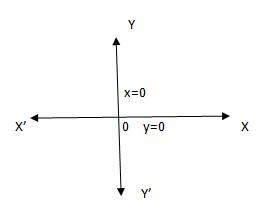QuestionAnswers

# Draw the graph of the equation x=0, y=0.Verified
155.4k+ views
Hint: The equations x=0 represents y -axis and y=0 represents x –axis.